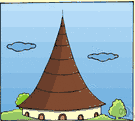# conic section

(redirected from Conic Sections in Polar Coordinates)
Also found in: Thesaurus, Encyclopedia.

## conic section

n.
1. The intersection of a right circular cone and a plane, which generates one of a group of plane curves, including the circle, ellipse, hyperbola, and parabola.
2. A graph of the general quadratic equation in two variables.

## conic section

n
(Mathematics) one of a group of curves formed by the intersection of a plane and a right circular cone. It is either a circle, ellipse, parabola, or hyperbola, depending on the eccentricity, e, which is constant for a particular curve e = 0 for a circle; e<1 for an ellipse; e = 1 for a parabola; e>1 for a hyperbola. Often shortened to: conic

## con′ic sec′tion

n.
a curve formed by the intersection of a plane with a right circular cone; an ellipse, a circle, a parabola, or a hyperbola. Also called conic.
[1655–65]

## conic section

A curve formed by the intersection of a plane with a cone. Conic sections can appear as circles, ellipses, hyperbolas, or parabolas, depending on the angle of the intersecting plane relative to the cone's base.
ThesaurusAntonymsRelated WordsSynonymsLegend:
 Noun 1conic section - (geometry) a curve generated by the intersection of a plane and a circular coneconicgeometry - the pure mathematics of points and lines and curves and surfacesplane figure, two-dimensional figure - a two-dimensional shapeellipse, oval - a closed plane curve resulting from the intersection of a circular cone and a plane cutting completely through it; "the sums of the distances from the foci to any point on an ellipse is constant"parabola - a plane curve formed by the intersection of a right circular cone and a plane parallel to an element of the curvehyperbola - an open curve formed by a plane that cuts the base of a right circular cone
Translations

## conic section

n (Math) → Kegelschnitt m
Site: Follow: Share:
Open / Close